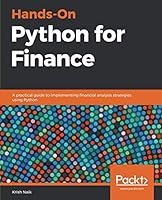# Hands-On Python for Finance0 Reviews
2019-03-30
378 pages

## Book Description

Learn and implement quantitative using popular Python libraries like NumPy, pandas, and Keras

#### Key Features

• Understand Python data structure fundamentals and work with time series data
• Use popular Python libraries including TensorFlow, Keras, and SciPy to deploy key concepts in quantitative finance
• Explore various Python programs and learn finance paradigms

#### Book Description

Python is one of the most popular languages used for quantitative finance. With this book, you'll explore the key characteristics of Python for finance, solve problems in finance, and understand .

The book starts with major concepts and techniques related to quantitative finance, and an introduction to some key Python libraries. Next, you'll implement time series analysis using pandas and DataFrames. The following chapters will help you gain an understanding of how to measure the diversifiable and non-diversifiable security risk of a portfolio and optimize your portfolio by implementing Markowitz Portfolio Optimization. Sections on regression analysis methodology will help you to value assets and understand the relationship between commodity prices and stocks. In addition to this, you'll be able to forecast stock prices using Monte Carlo simulation. The book will also highlight forecast models that will show you how to determine the price of a call option by analyzing price variation. You'll also use deep learning for financial and forecasting. In the concluding chapters, you will create neural with TensorFlow and Keras for forecasting and prediction.

By the end of this book, you will be equipped with the skills you need to perform different financial analysis tasks using Python

#### What you will learn

• Clean financial data with data preprocessing
• Visualize financial data using histograms, color plots, and graphs
• Perform time series analysis with pandas for forecasting
• Estimate covariance and the correlation between securities and stocks
• Optimize your portfolio to understand risks when there is a possibility of higher returns
• Calculate expected returns of a stock to measure the performance of a portfolio
• Create a prediction model using recurrent neural networks (RNN) with Keras and TensorFlow

#### Who this book is for

This book is ideal for aspiring data scientists, Python developers and anyone who wants to start performing quantitative finance using Python. You can also make this beginner-level guide your first choice if you're looking to pursue a career as a financial analyst or a data analyst. Working knowledge of Python programming language is necessary.

1. Python for Finance 101
2. Getting started with Numpy, Pandas and Matplotlib
3. Time Series Analysis and Forecasting
4. Measuring Investment Risks
5. Portfolio Allocation and Markowitz Portfolio Optimization
6. The Capital Asset Pricing Model
7. Regression Analysis in Finance
8. Monte Carlo simulations for decision-making
9. Option Pricing-Black Scholes Model
10. Introduction to Deep Learning with TensorFlow and Keras
11. Stock Market Analysis and Forecasting Case study
12. What Is Next

## Book Details

• Title: Hands-On Python for Finance
• Author:
• Length: 378 pages
• Edition: 1
• Language: English
• Publisher:
• Publication Date: 2019-03-30
• ISBN-10: 1789346371
• ISBN-13: 9781789346374